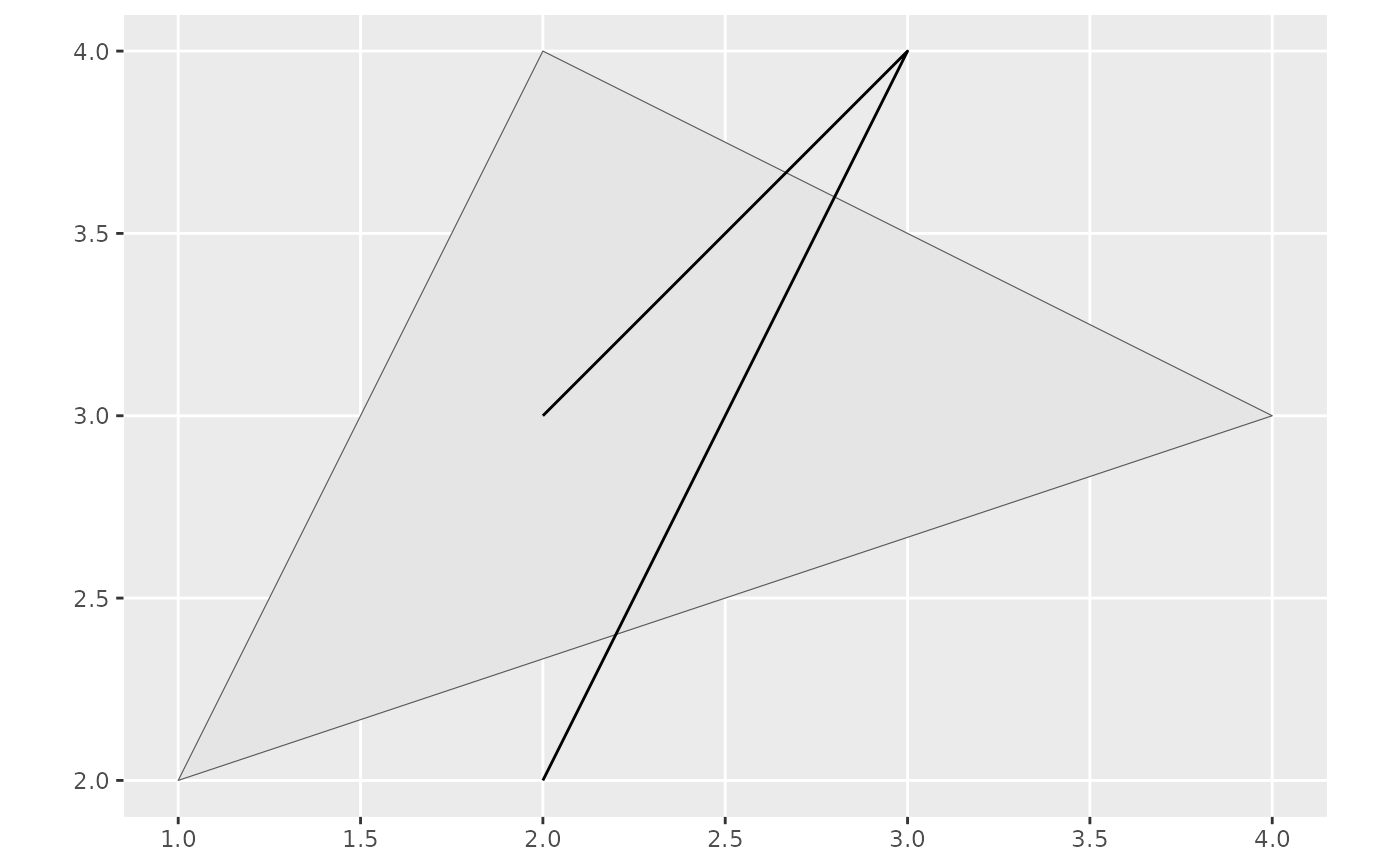geom_fm is a generic function for generating geomes from various kinds of fmesher objects, e.g. fm_segm and fm_mesh_2d. The function invokes particular methods which depend on the class of the data argument. Requires the ggplot2 package.

Note: geom_fm is not yet a "proper" ggplot2 geom method; the interface may therefore change in the future.

## Usage

geom_fm(mapping = NULL, data = NULL, ...)

# S3 method for fm_mesh_2d
geom_fm(
mapping = NULL,
data = NULL,
mapping_int = NULL,
mapping_bnd = NULL,
defs_int = NULL,
defs_bnd = NULL,
...,
crs = NULL
)

# S3 method for fm_segm
geom_fm(mapping = NULL, data = NULL, ..., crs = NULL)

# S3 method for fm_mesh_1d
geom_fm(
mapping = NULL,
data = NULL,
...,
xlim = NULL,
basis = TRUE,
knots = TRUE,
derivatives = FALSE,
weights = NULL
)

## Arguments

mapping

an object for which to generate a geom.

data

an object for which to generate a geom.

...

Arguments passed on to the geom method.

mapping_int

aes for interior constraint edges.

mapping_bnd

aes for boundary edges.

defs_int

additional settings for interior constraint edges.

defs_bnd

crs

Optional crs to transform the object to before plotting.

xlim

numeric 2-vector; specifies the interval for which to compute functions. Default is data$interval basis logical; if TRUE (default), show the spline basis functions knots logical; if TRUE (default), show the spline knot locations derivatives logical; if TRUE (not default), draw first order derivatives instead of function values weights numeric vector; if provided, draw weighted basis functions and the resulting weighted sum. ## Value A combination of ggplot2 geoms. ## Methods (by class) • geom_fm(fm_mesh_2d): Converts an fm_mesh_2d() object to sf with fm_as_sfc() and uses geom_sf to visualize the triangles and edges. • geom_fm(fm_segm): Converts an fm_segm() object to sf with fm_as_sfc() and uses geom_sf to visualize it. • geom_fm(fm_mesh_1d): Evaluates and plots the basis functions defined by an fm_mesh_1d() object. ## Examples ggplot() + geom_fm(data = fmexample$mesh)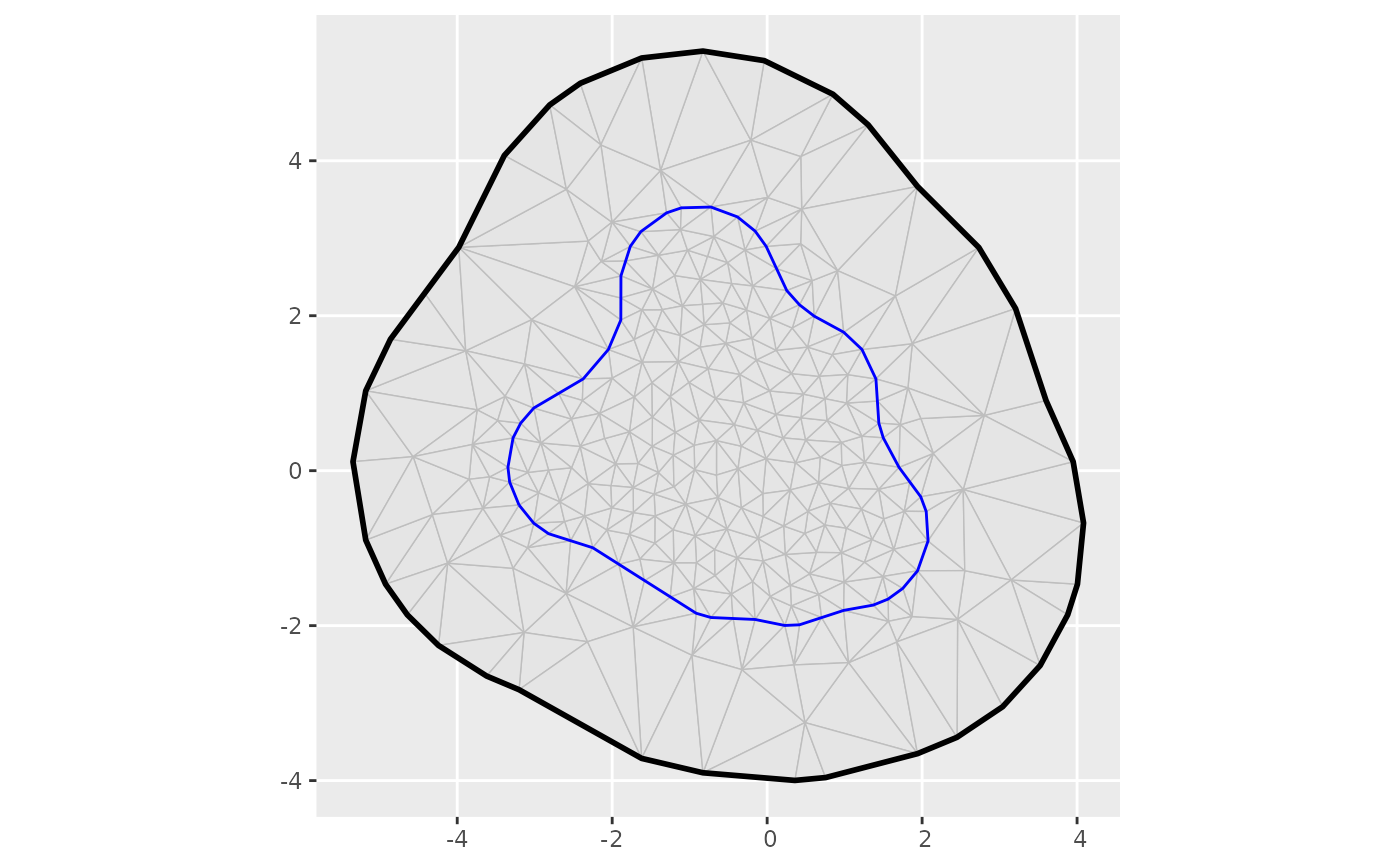m <- fm_mesh_2d(
cbind(10, 20),
boundary = fm_extensions(cbind(10, 20), c(25, 65)),
max.edge = c(4, 10),
crs = fm_crs("+proj=longlat")
)
ggplot() +
geom_fm(data = m)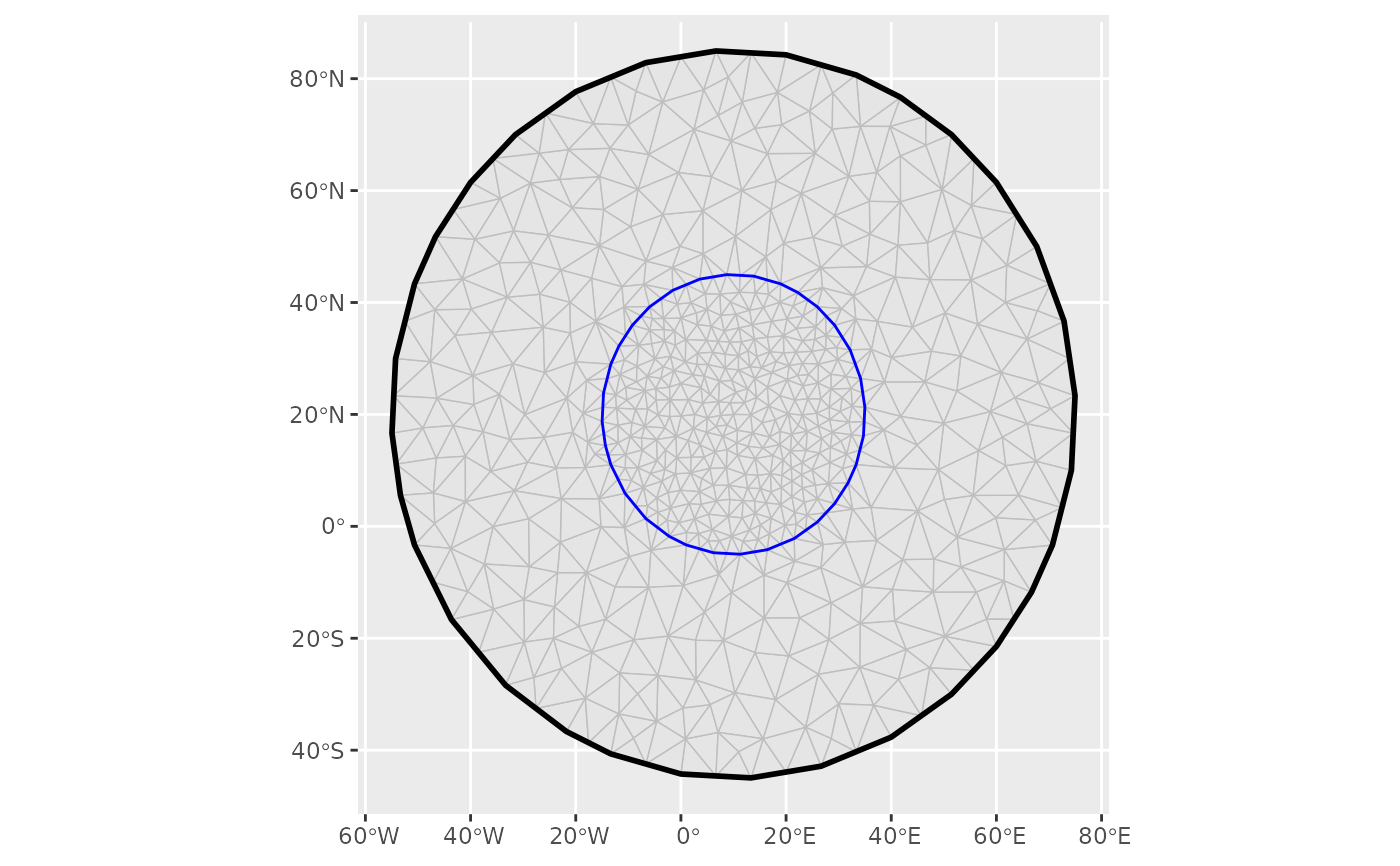ggplot() +
geom_fm(data = m, crs = fm_crs("epsg:27700"))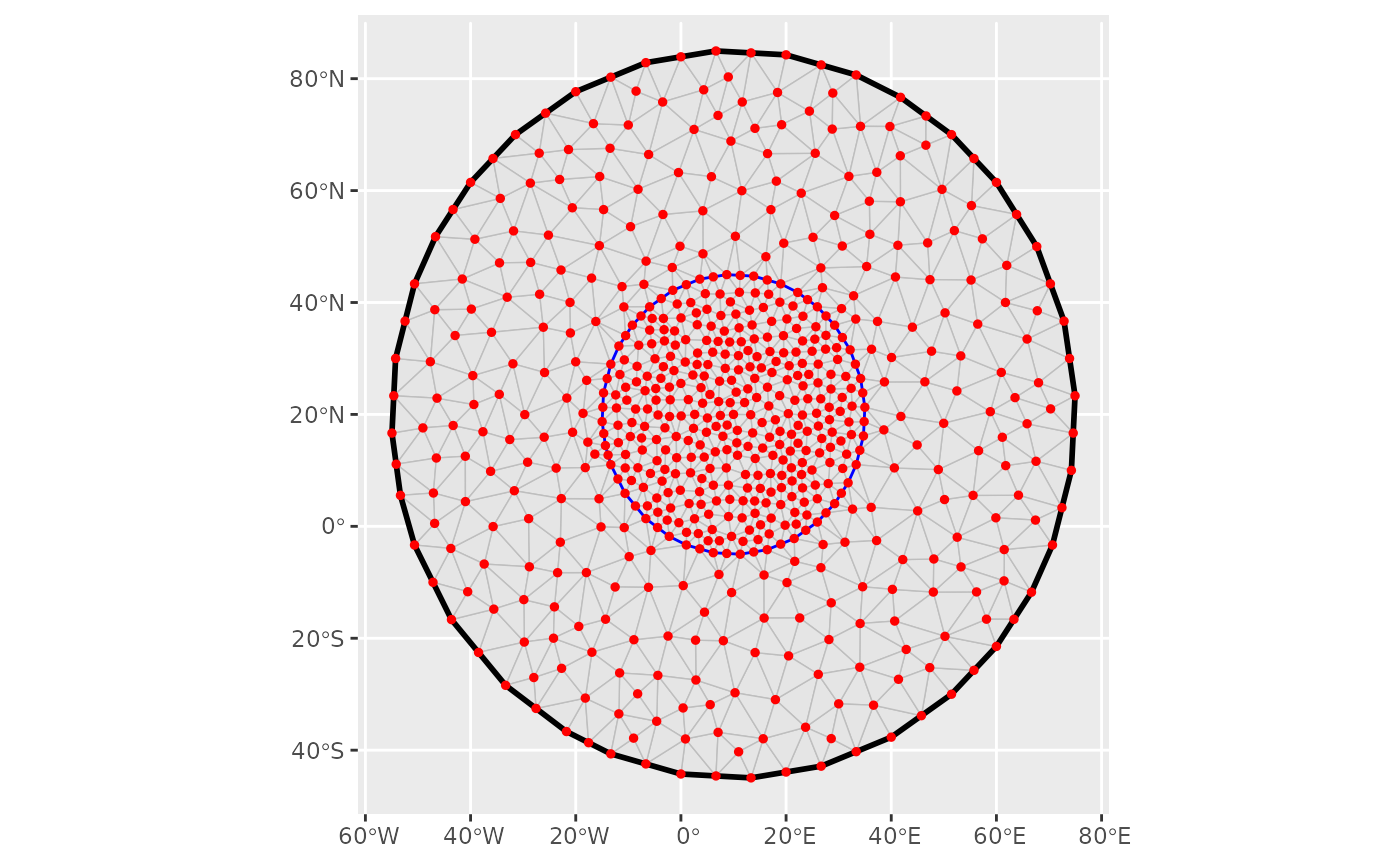# \donttest{
# Compute a mesh vertex based function on a different grid
px <- fm_pixels(fm_transform(m, fm_crs("mollweide_globe")))
px$fun <- fm_evaluate(m, loc = px, field = sin(m$loc[, 1] / 5) * sin(m\$loc[, 2] / 5)
)
ggplot() +
geom_tile(aes(geometry = geometry, fill = fun),
data = px,
stat = "sf_coordinates"
) +
geom_fm(
data = m, alpha = 0.2, linewidth = 0.05,
crs = fm_crs("mollweide_globe")
)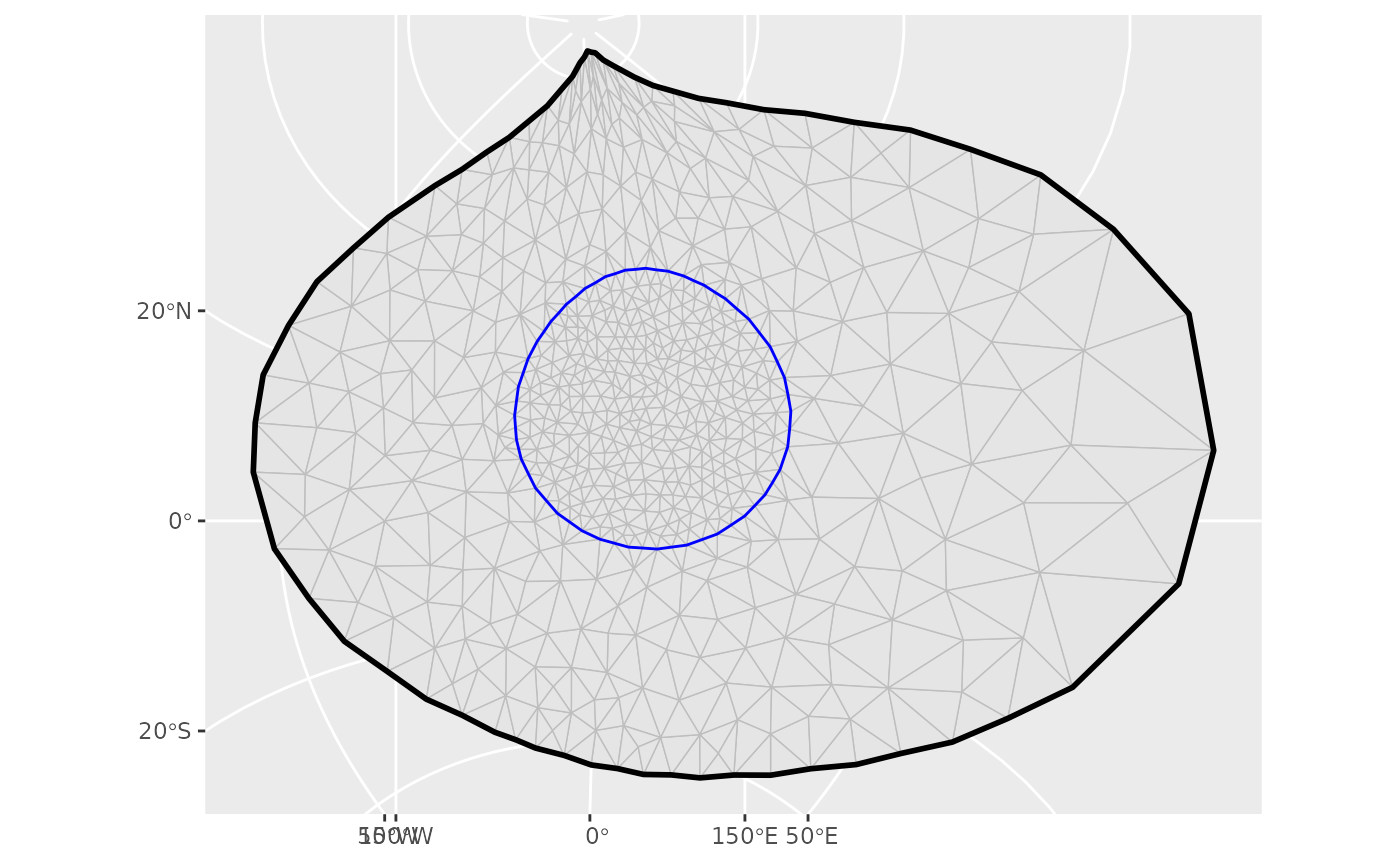# }
m <- fm_mesh_1d(c(1, 2, 4, 6, 10), boundary = c("n", "d"), degree = 2)
ggplot() +
geom_fm(data = m, weights = c(4, 2, 4, -1))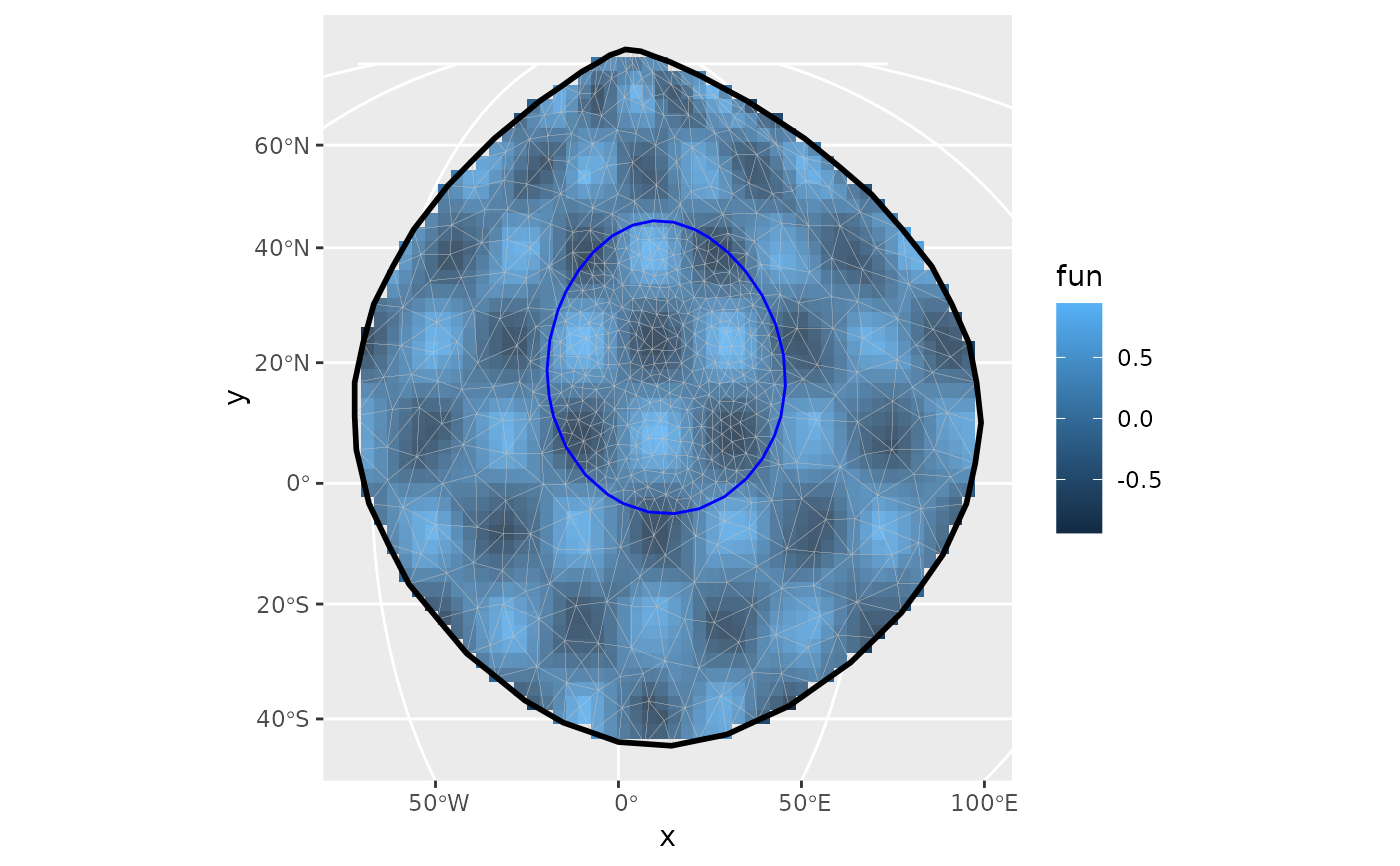m <- fm_mesh_1d(
c(1, 2, 3, 5, 7),
boundary = c("dirichlet", "neumann"),
degree = 2
)
ggplot() +
geom_fm(data = m)